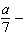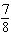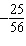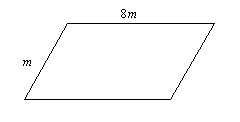Name:    Quiz 2.4-2.6

Multiple Choice
Identify the choice that best completes the statement or answers the question.

Solve the equation. Check your solution.

1.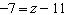a. –18 c. 0.64 b. 4 d. –4

2.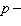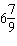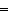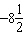a.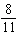c.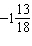b.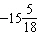d.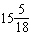3.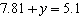a. 2.71 c. –2.71 b. 1.53 d. –12.91

4.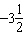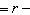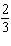a.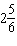c.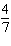b.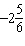d.Write the verbal sentence as an equation. Then solve.

5.

7 less than a number n is –9.
 a.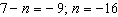c.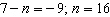b.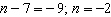d.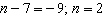Solve the equation. Check your solution.

6.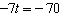a. –77 c. 10 b. 490 d. –63

7.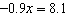a. 7.2 c. –7.29 b. 9 d. –9

8.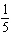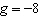a.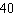c.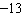b.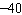d.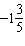9.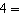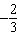a.c.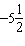b.d.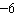10.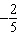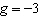a.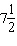c.b.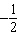d.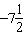Write the verbal sentence as an equation. Then solve.

11.

A number divided by –6 is –8.
 a.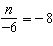; n =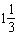c.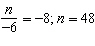b.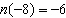; n = 48 d.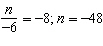Match the equation with the first step to solve it.

12.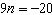a. Multiply by 9. c. Subtract 9. b. Divide by 9. d. Add 9.

Solve the equation. Check your solution.

13.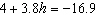a. –20.9 c. –5.5 b. –12.9 d. –20.7

Numeric Response

Solve the equation. Check your solution.

1.